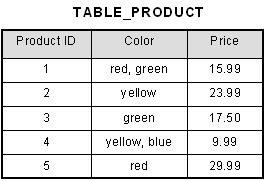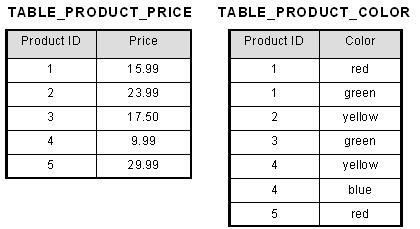# First Normal Form (1NF)

## 1st Normal Form Definition

A database is in first normal form if it satisfies the following conditions:

• Contains only atomic values
• There are no repeating groups

An atomic value is a value that cannot be divided. For example, in the table shown below, the values in the [Color] column in the first row can be divided into "red" and "green", hence [TABLE_PRODUCT] is not in 1NF.

A repeating group means that a table contains two or more columns that are closely related. For example, a table that records data on a book and its author(s) with the following columns: [Book ID], [Author 1], [Author 2], [Author 3] is not in 1NF because [Author 1], [Author 2], and [Author 3] are all repeating the same attribute.

## 1st Normal Form Example

How do we bring an unnormalized table into first normal form? Consider the following example:This table is not in first normal form because the [Color] column can contain multiple values. For example, the first row includes values "red" and "green."

To bring this table to first normal form, we split the table into two tables and now we have the resulting tables:Now first normal form is satisfied, as the columns on each table all hold just one value.